# Efficient C Code C code Compiler Machine code

• Slides: 22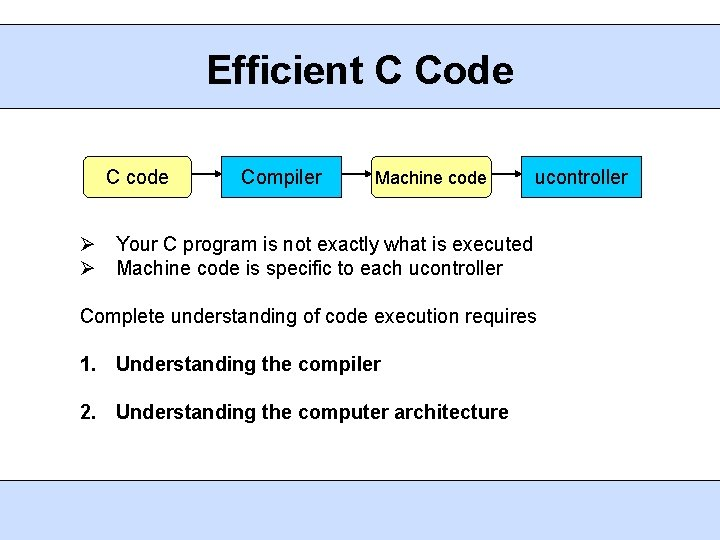Efficient C Code C code Compiler Machine code ucontroller Your C program is not exactly what is executed Machine code is specific to each ucontroller Complete understanding of code execution requires 1. Understanding the compiler 2. Understanding the computer architectureARM Instruction Set An instruction set is the set of all machine instructions supported by the architecture Load-Store Architecture • Data processing occurs in registers • Load and store instructions move data between memory and registers • [] indicate an address Ex. LDR r 0, [r 1] moves data into r 0 from memory at address in r 1 STR r 0, [r 1] moves data from r 0 into memory at address in r 1Data Processing Instructions Move Instructions MOV r 0, r 1 moves the contents of r 1 into r 0 MOV r 0, #3 moves the number 3 into r 0 Shift Instructions – inputs to operations can be shifted MOV r 0, r 1, LSL #2 moves (r 1 << 2) into r 0 MOV r 0, r 1, ASR #2 moves (r 1 >> 2) into r 0, sign extend Arithmetic Instructions ADD r 3, r 4, r 5 places (r 4 + r 5) in r 3Condition Flags Current Program Status Register (CPSR) contains the status of comparison instructions and some arithmetic instructions N – negative, Z – zero, C – unsigned carry, V – overflow, Q saturation Flags are set as a result of a comparison instruction or an arithmetic instruction with an 'S' suffix Ex. CMP r 0, r 1 – sets status bits as a result of (r 0 – r 1) ADDS r 0, r 1, r 2 – r 0 = r 1 + r 2 and status bits set ADD r 0, r 1, r 2 – r 0 = r 1 + r 2 but no status bits set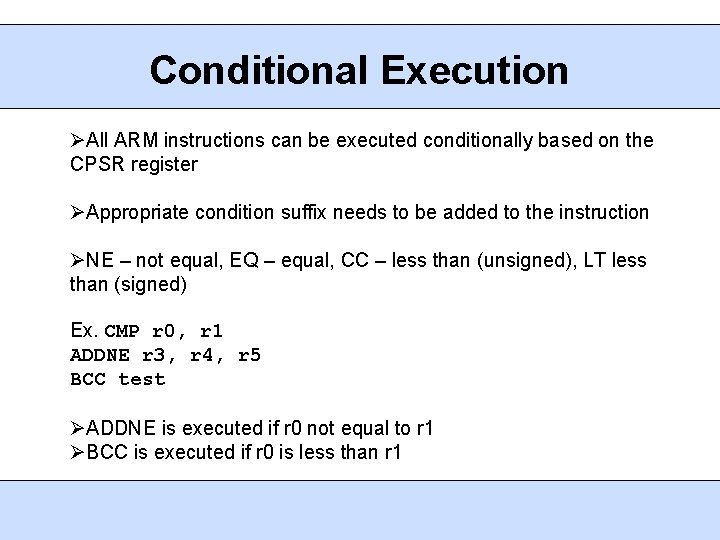Conditional Execution All ARM instructions can be executed conditionally based on the CPSR register Appropriate condition suffix needs to be added to the instruction NE – not equal, EQ – equal, CC – less than (unsigned), LT less than (signed) Ex. CMP r 0, r 1 ADDNE r 3, r 4, r 5 BCC test ADDNE is executed if r 0 not equal to r 1 BCC is executed if r 0 is less than r 1Variable Types and Casting Program computes the sum of the first 64 elts in the data array Variable i is declared as a char to save space int checksum_v 1 (int *data) { char i; int sum=0; for (i=0; i<64; i++) { sum += data[I]; } return sum; } i always less than 8 bits long May use less register space and/or stack space i as a char does NOT save any space All stack entries and registers are 32 bits long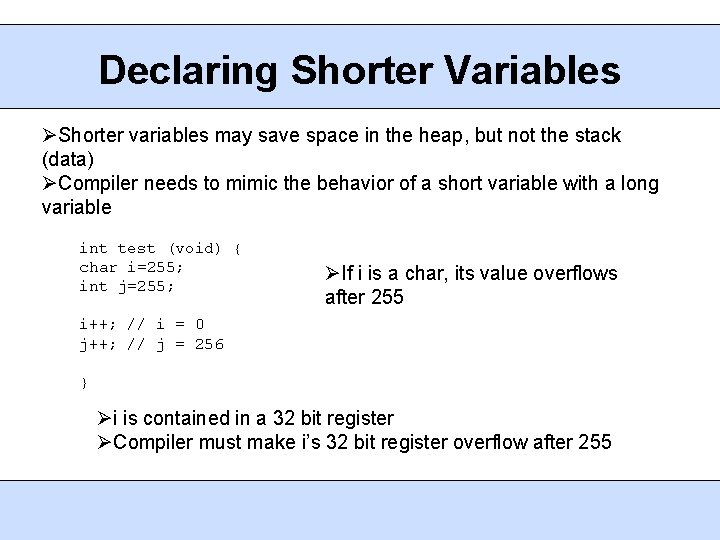Declaring Shorter Variables Shorter variables may save space in the heap, but not the stack (data) Compiler needs to mimic the behavior of a short variable with a long variable int test (void) { char i=255; int j=255; If i is a char, its value overflows after 255 i++; // i = 0 j++; // j = 256 } i is contained in a 32 bit register Compiler must make i’s 32 bit register overflow after 255Assembly Code for Checksum checksum_v 1 MOV r 2, r 0 ; r 2 = data MOV r 0, #0 ; sum = 0 MOV r 1, #0 ; i = 0 checksum_v 1_loop LDR r 3, [r 2, r 1, LSL #2] ; r 3 = data[I] ADD r 1, #1 ; r 1 = i+1 AND r 1, #0 xff ; i = (char)r 1 CMP r 1, #0 x 40 ; compare i, 64 ADD r 0, r 3, r 0 ; sum += r 3 BCC checksum_v 1_loop ; if i<64 goto loop MOV pc, r 14 • Argument, *data, passed in r 0 • Return address stored in r 14 • Stack avoided to reduce delay • LSL needed to increment by 4 • Highlighted instruction needed to mimic char • 17% instruction overhead Declaring i as an unsigned int would fix the problem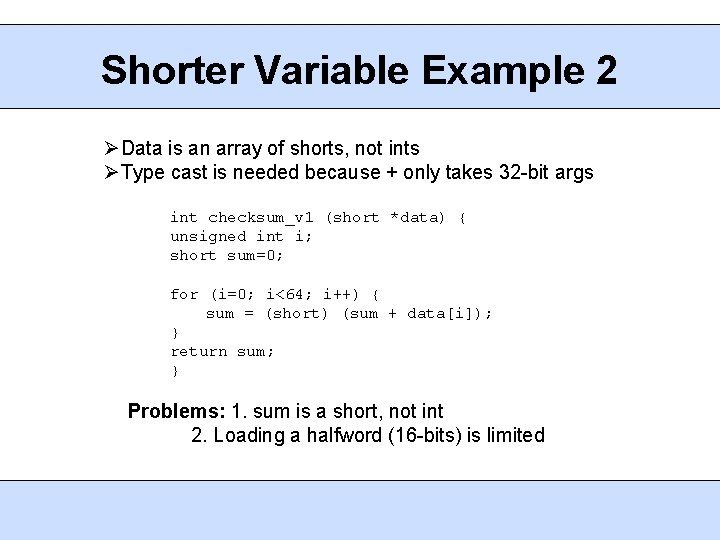Shorter Variable Example 2 Data is an array of shorts, not ints Type cast is needed because + only takes 32 -bit args int checksum_v 1 (short *data) { unsigned int i; short sum=0; for (i=0; i<64; i++) { sum = (short) (sum + data[i]); } return sum; } Problems: 1. sum is a short, not int 2. Loading a halfword (16 -bits) is limited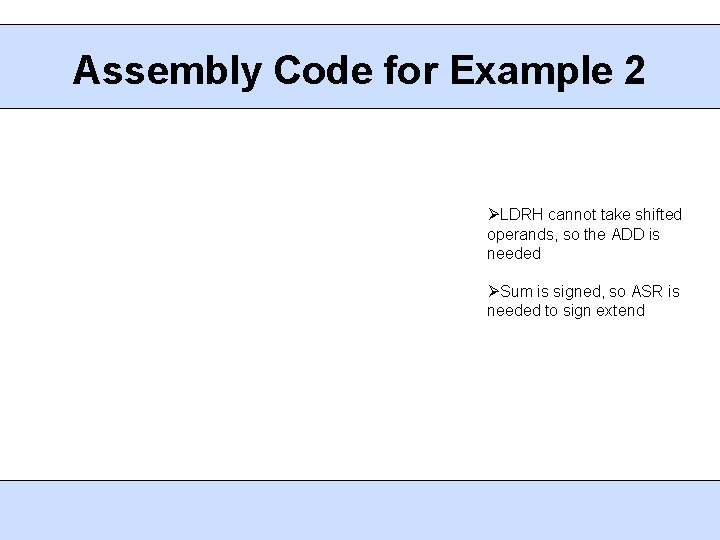Assembly Code for Example 2 LDRH cannot take shifted operands, so the ADD is needed Sum is signed, so ASR is needed to sign extend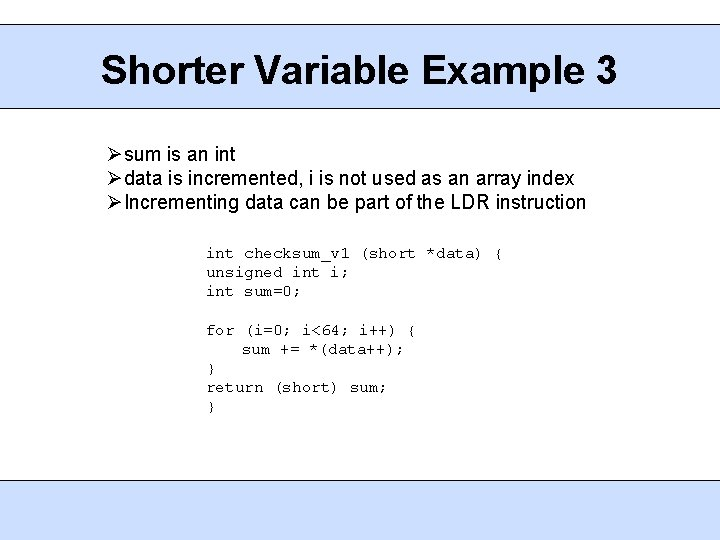Shorter Variable Example 3 sum is an int data is incremented, i is not used as an array index Incrementing data can be part of the LDR instruction int checksum_v 1 (short *data) { unsigned int i; int sum=0; for (i=0; i<64; i++) { sum += *(data++); } return (short) sum; }Assembly Code for Example 3 checksum_v 1 MOV r 2, #0 MOV r 1, #0 checksum_v 1_loop LDRSH r 3, [r 0], #2 ADD r 1, #1 CMP r 1, #0 x 40 ADD r 2, r 3, r 2 BCC checksum_v 1_loop MOV r 0, r 2, LSL #16 MOV r 0, ASR #16 MOV pc, r 14 ; sum = 0 ; i = 0 ; ; ; r 3 = *(data++) r 1 = i+1 compare i, 64 sum += r 3 if i<64 goto loop ; r 0 = (short)sum ; return sum *data is incremented as part of LDRSH instruction Cast to short occurs once, outside of the loop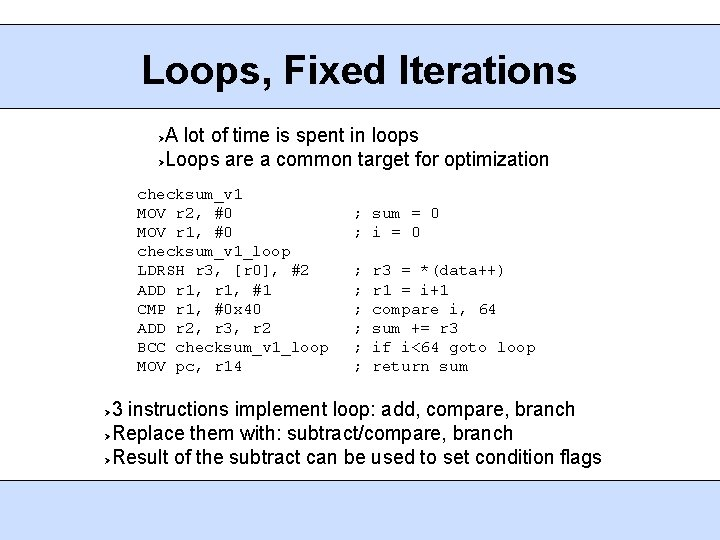Loops, Fixed Iterations A lot of time is spent in loops Loops are a common target for optimization checksum_v 1 MOV r 2, #0 MOV r 1, #0 checksum_v 1_loop LDRSH r 3, [r 0], #2 ADD r 1, #1 CMP r 1, #0 x 40 ADD r 2, r 3, r 2 BCC checksum_v 1_loop MOV pc, r 14 ; sum = 0 ; i = 0 ; ; ; r 3 = *(data++) r 1 = i+1 compare i, 64 sum += r 3 if i<64 goto loop return sum 3 instructions implement loop: add, compare, branch Replace them with: subtract/compare, branch Result of the subtract can be used to set condition flags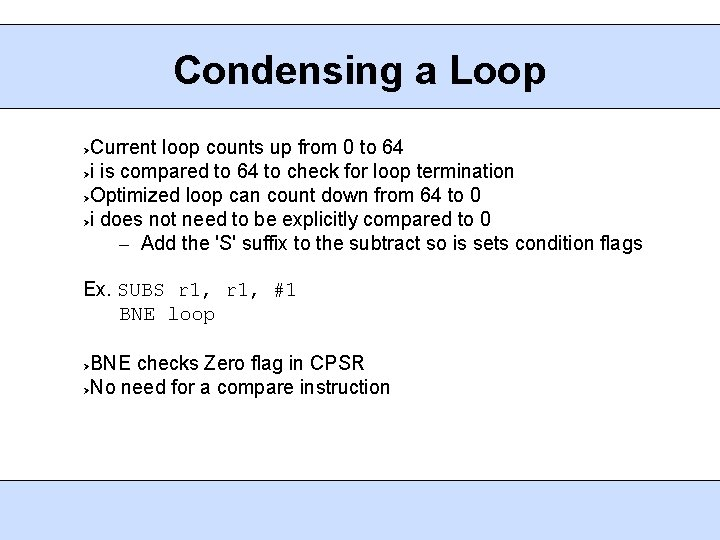Condensing a Loop Current loop counts up from 0 to 64 i is compared to 64 to check for loop termination Optimized loop can count down from 64 to 0 i does not need to be explicitly compared to 0 – Add the 'S' suffix to the subtract so is sets condition flags Ex. SUBS r 1, #1 BNE loop BNE checks Zero flag in CPSR No need for a compare instructionLoops, Counting Down checksum MOV r 2, r 0 MOV r 0, #0 MOV r 1, #0 x 40 checksum_loop LDR r 3, [r 2], #4 SUBS r 1, #1 ADD r 0, r 3, r 0 BCC checksum_loop MOV pc, r 14 ; r 2 = data ; sum = 0 ; i = 64 ; r 3 = *(data++) ; i-- and set flags ; sum += r 3 ; if i!=0 goto loop ; return sum One comparison instruction removed from inside the loop Possible because ARM always compares to 0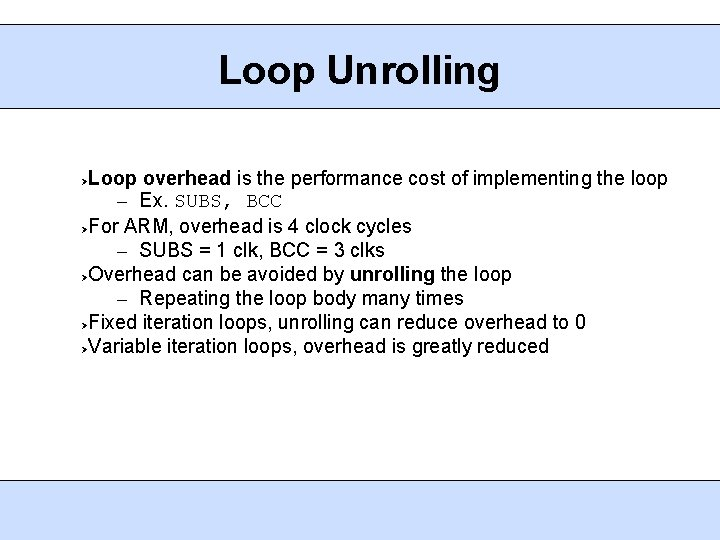Loop Unrolling Loop overhead is the performance cost of implementing the loop – Ex. SUBS, BCC For ARM, overhead is 4 clock cycles – SUBS = 1 clk, BCC = 3 clks Overhead can be avoided by unrolling the loop – Repeating the loop body many times Fixed iteration loops, unrolling can reduce overhead to 0 Variable iteration loops, overhead is greatly reduced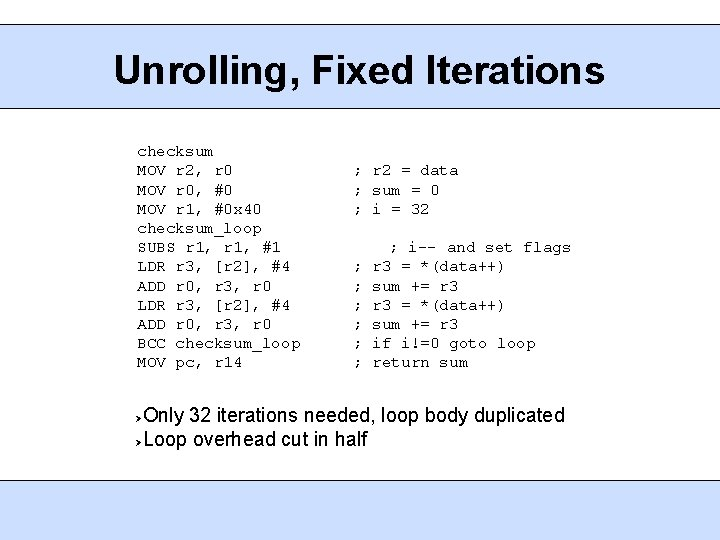Unrolling, Fixed Iterations checksum MOV r 2, r 0 MOV r 0, #0 MOV r 1, #0 x 40 checksum_loop SUBS r 1, #1 LDR r 3, [r 2], #4 ADD r 0, r 3, r 0 BCC checksum_loop MOV pc, r 14 ; r 2 = data ; sum = 0 ; i = 32 ; ; ; ; i-- and set flags r 3 = *(data++) sum += r 3 if i!=0 goto loop return sum Only 32 iterations needed, loop body duplicated Loop overhead cut in half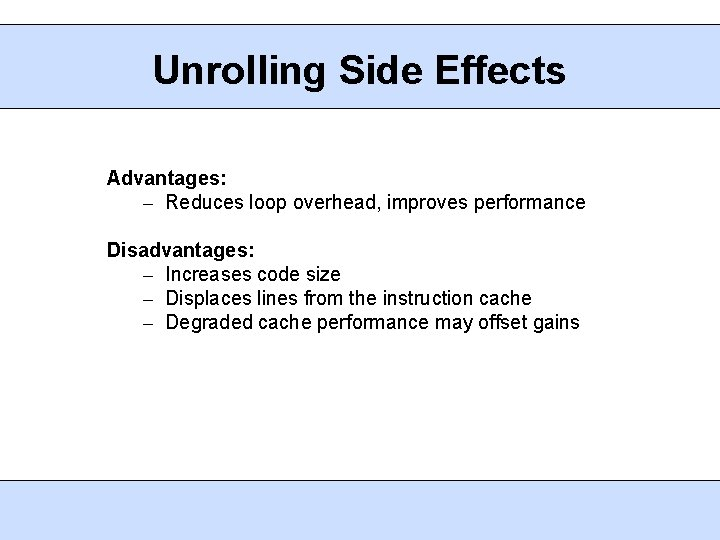Unrolling Side Effects Advantages: – Reduces loop overhead, improves performance Disadvantages: – Increases code size – Displaces lines from the instruction cache – Degraded cache performance may offset gains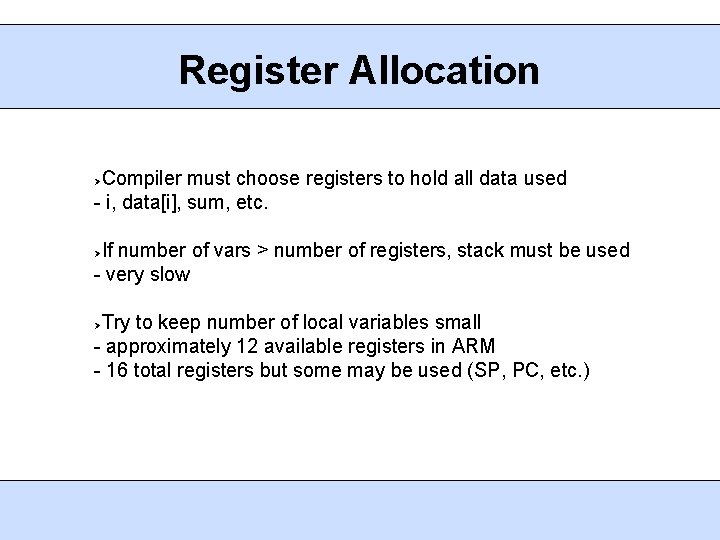Register Allocation Compiler must choose registers to hold all data used - i, data[i], sum, etc. If number of vars > number of registers, stack must be used - very slow Try to keep number of local variables small - approximately 12 available registers in ARM - 16 total registers but some may be used (SP, PC, etc. )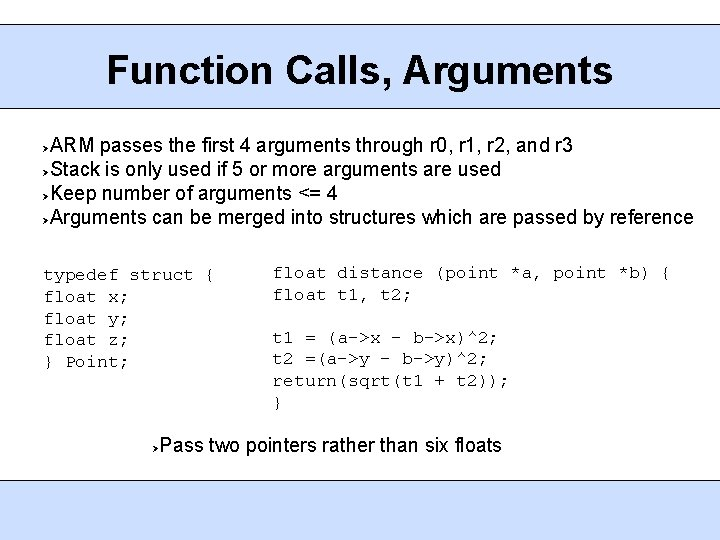Function Calls, Arguments ARM passes the first 4 arguments through r 0, r 1, r 2, and r 3 Stack is only used if 5 or more arguments are used Keep number of arguments <= 4 Arguments can be merged into structures which are passed by reference typedef struct { float x; float y; float z; } Point; float distance (point *a, point *b) { float t 1, t 2; t 1 = (a->x – b->x)^2; t 2 =(a->y – b->y)^2; return(sqrt(t 1 + t 2)); } Pass two pointers rather than six floatsPreserving Registers Caller must preserve registers that the callee might corrupt Registers are preserved by writing them to memory and reading them back later Example: – Function foo() calls function bar() – Both foo() and bar() use r 4 and r 5 – Before the call, foo() writes registers to memory (STR) – After the call, foo() reads memory back (LDR) If foo() and bar() are in different. c files, compiler will preserve all corruptible registers If foo() and bar() are in the same file, compiler will only save corrupted registers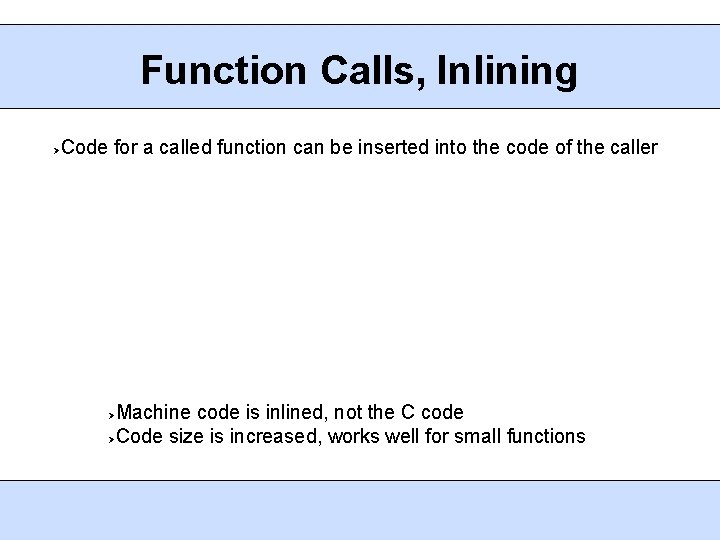Function Calls, Inlining Code for a called function can be inserted into the code of the caller Machine code is inlined, not the C code Code size is increased, works well for small functions